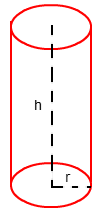# Surface Area of a Cylinder Formula

The surface area of a cylinder formula is given here along with the explanation and definition of the surface area of a cylinder. An example question based on the surface area formula for a cylinder is also given which can help to understand the concept in a better way. Also, check out all the cylinder formula to know more about the TSA, curved area, and volume of a cylinder.

## Surface Area of a Cylinder Definition

A solid geometrical structure that has two circular bases and two parallel faces then it is cylinder. The circular base radius is also the radius of the cylinder and the of the parallel faces if considered as the height of the cylinder. The total area occupied by the surface of the cylinder is the surface area of the cylinder. The unit of measurement of the surface area is in square units.## Surface Area of a Cylinder Formula

The Surface Area of a Cylinder Formula is

Formula for Surface Areas of Cylinders
Total Surface Area of a Cylinder TSA = 2 π r(r+h)
Curved Surface Area of a Cylinder CSA = 2 π r h

Where

• r is the radius of the circular base of the cylinder
• h is the height of the parallel face of the cylinder

### Examples Question Based on Surface Area of a Cylinder

Question 1: What will be the surface of the cylinder with height 10 cm and the diameter of the base is 12 cm.

Solution:

Given, Height = 10 cm Diameter = 12 cm

Hence, Radius = d/2 = 12/2 = 6 cm

According to the formula:

TSA = 2πr(r+h)

2×3.14×6(6+10)

=602.88cm2

Stay tuned with BYJU’S for more math formulas on different maths topics and learn various other maths concepts easily from several engaging video lessons by registering now.